# 双指针判断单链表是否有环的正确性证明

​问题：给你一个单链表，需要找到一个方法进行判断是否有环的存在。

## 双指针判断单链表是否有环

​使用两个slow, fast指针从头开始扫描链表。指针slow 每次走1步，指针fast每次走2步。如果存在环，则指针slow、fast会相遇；如果不存在环，指针fast遇到NULL退出。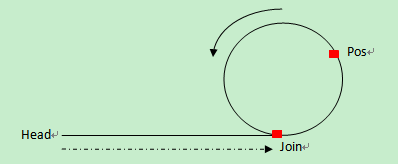## 证明存在环就会导致两个指针（slow和fast指针）一定相遇。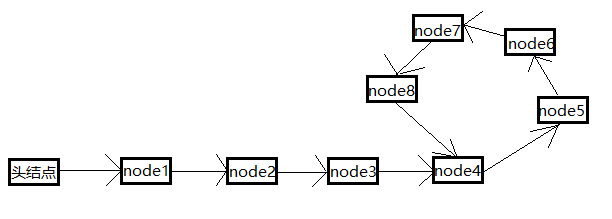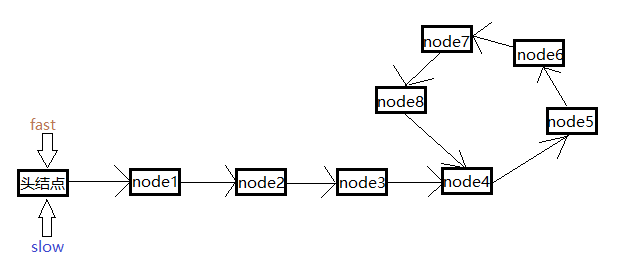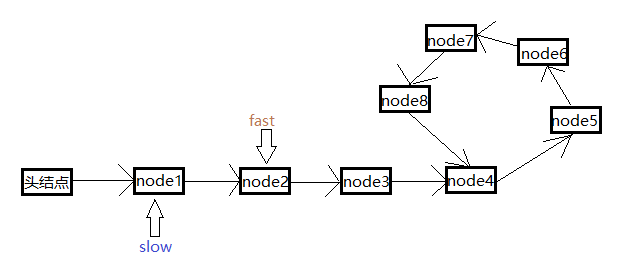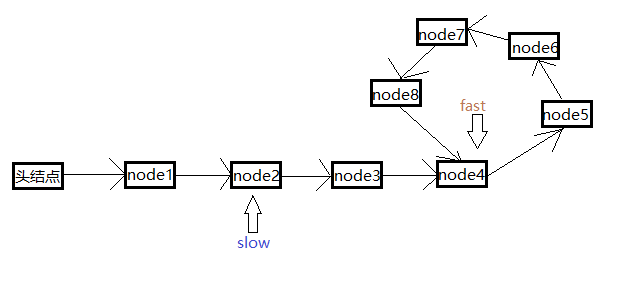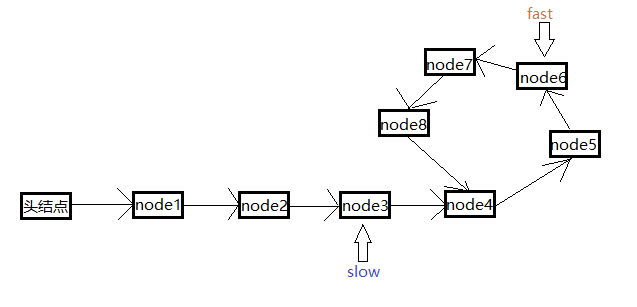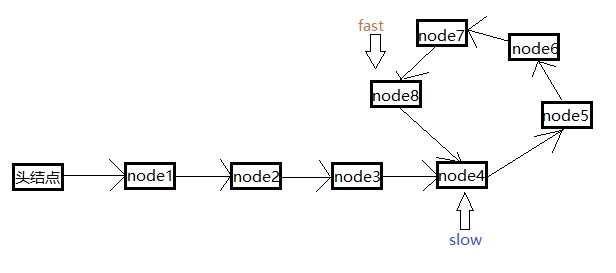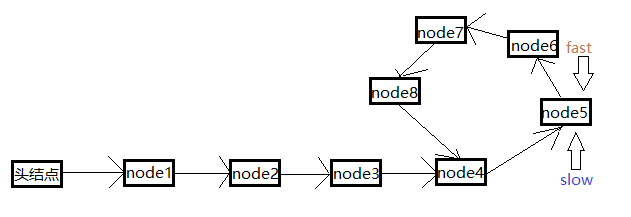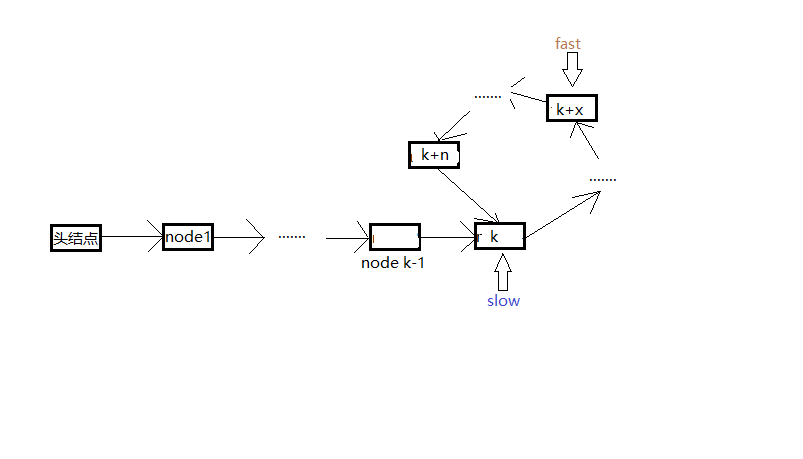(1) slow指针每次移动一个节点，到达k号节点，刚好是进入环的节点。
(2) fast节点每次移动二个节点，通过2*k个节点，到达k+x号节点，处于环中。

$x =（2*k -k）\%（n+1）$

\begin{aligned} m=&n-x+1\\ =&n+1-(2*k -k)\%(n+1) \\ =&n+1 - k\%(n+1) \end{aligned}

m满足：

$m<(n+1)$

$k+m$
fast节点会到达：

\begin{aligned} k+(x+2*m)\%(n+1)=&k+((2*k -k )\%(n+1) + 2*m)\%(n+1) \\ =&k+(k\%(n+1)+ 2*((n+1) - k\%(n+1)))\%(n+1) \\ =&k+(k\%(n+1) + 2*(n+1) - 2*k\%(n+1))\%(n+1) \\ =&k+(2*(n+1)-k\%(n+1))\%(n+1) \\ =&k+(n+1 - k\%(n+1))\%(n+1)+(n+1)\%(n+1) \\ =&k+(n+1 - k\%(n+1))\%(n+1) \end{aligned}

\begin{aligned} \because \quad &m=n+1-k\%(n+1) \\ \therefore \quad &k+(x+2*m)\%(n+1)=k+m\%(n+1) \\ \because \quad &m<(n+1) \\ \therefore \quad &m\%(n+1)=m \\ \therefore \quad &k+(x+2*m)\%(n+1)=k+m \end{aligned}

## 综上所述：

slow指针进入环后，只要再走m步（fast落后slow指针的节点数）就一定会和fast指针相遇。slow指针进入环后，假设fast指针再往前走m个节点就会到达当前slow指针的位置，那么当slow指针和fast指针同时移动的时候，slow指针每向前移动一个节点，fast指针就会向前移动两个节点，fast与slow之间的距离就会缩短一个，所以当移动m步之后，fast指针就会和slow指针相遇。